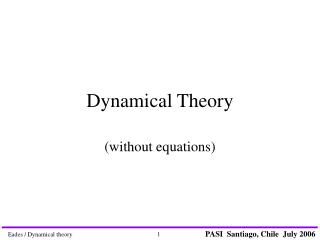DownloadDownload PresentationDynamical Theory

# Dynamical Theory

Télécharger la présentation## Dynamical Theory

- - - - - - - - - - - - - - - - - - - - - - - - - - - E N D - - - - - - - - - - - - - - - - - - - - - - - - - - -
##### Presentation Transcript

1. Dynamical Theory (without equations)

2. Dynamical Theory • This lecture aims to communicate some intuitive bases for thinking dynamically • Some familiarity with basic ideas of diffraction (for example, Bragg’s law and the reciprocal lattice) will be assumed.

3. Kinematical vs Dynamical theory. • Any sample more than a few atoms thick requires dynamical theory. • A simple argument suggests that if the sample thickness is greater than about ξg/8, then dynamical theory is necessary

4. Bragg’s Law Bragg’s Law 2d sin θ = λ still tells us where there are diffracted beams.

5. Rocking Curves How far from the Bragg angle do we get diffraction? For thick samples Δθ = 4/gξg ξg is the extinction distance For thin samples Δθ = 1/gt t is the sample thickness

6. Reimer Transmission Electron Microscopy

7. Channeling Electrons do not travel through crystals with a uniform distribution. When electrons are incident on a crystal close to the Bragg angle, the electrons are channeled down the atomic planes. Indeed, Bragg reflection may be thought of as the result of electrons coupling into channeling states.

8. Channeling II The channeling states are also called Bloch states or Bloch waves. Bloch waves consist of electrons traveling parallel to the planes but the electrons are not uniformly distributed. The Bloch waves may be channeled onto the atoms or between the atoms. Defect contrast arises because the defects scatter electrons from one Bloch state to another.

9. To understand dynamical diffraction it is convenient to use a new graphical construction. We replace the Ewald sphere construction with a dispersion surface construction. For kinematical diffraction they give the same result but it is easier to extend the dispersion surface construction to describe dynamical diffraction

10. Constructions

11. End • Dynamical diffraction explains everything.

12. Two-beam dynamical